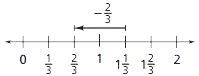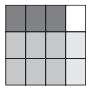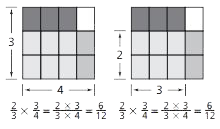Toggle Accessibility Tools

# 6-3 Let's Be Rational - Concepts and Explanations

## Addition and Subtraction of Fractions

Students model and symbolize problems to develop meaning and skill in addition and subtraction.

Students find common denominators so that the numerators can be added or subtracted.

### Example

To find the sum of A + B, or 1/2 + 1/8, on the rectangle, students need to use equivalent fractions to rename 1/2 as 4/8. The area model helps students visualize A, 1/2, as 4 eighth-size sections, or 4/8. Students can write the number sentence 4/8+ 1/8 = 5/8 to see why they need to rename fractions when adding and subtracting.The number-line model helps students make the connection between fractions and numbers or quantities. This number line illustrates 1 1/32/3 = 2/3.## Developing the Multiplication Algorithm

Students notice that multiplication is easier for proper fractions than for other types of fractions because they can just multiply the numerators and multiply the denominators of the factors.

Models can support students in understanding why this works.

### Example

An area model can show 2/3 × 3/4. First, draw a square, cut it into 4 equivalent vertical strips, and shade 3/4 of the square. Next, to represent taking 2/3  of  3/4, cut the square into thirds the opposite way, and shade two of the three sections in a different color. The overlap of these shaded sections represents the product, 6/12.The denominators partition and repartition the whole. Breaking each of the fourths into three parts makes 12 pieces. When you multiply the denominators (3 × 4) in the algorithm, you resize the whole to have the correct number of parts.The numerator keeps track of how many of the parts are being referenced. You need to consider 2 out of 3 sections for each 1/4 of the square. This can be represented by the product of the numerators 2 × 3.

## Developing a Division Algorithm

As students work toward trying to develop and use algorithms, they may need to continue drawing pictures to help them think through the problems.

Our goal in the development of algorithms is to help students develop efficient algorithms. Students may have various ways to think about division of fractions.

### Example

Multiplying by the Denominator and Dividing by the Numerator: The reasoning for 9 ÷ 1/3 is: I have to find the total number of 1/3's in 9. There are three 1/3's in 1, so there must be 9 × 3 thirds in 9.  9 ÷ 1/3 = 9 × 3 = 27.

With 2/3 ÷ 3/4 = 2/3 x 4 ÷ 3, multiplying by 4 tells how many 1/4's are in a whole. Dividing by 3 adjusts this to account for grouping 3 of the 1/4's in this problem. Multiplying by denominator of the divisor, then dividing by the numerator is the same as multiplying by the reciprocal of the divisor. So, 2/3 ÷ 3/4 = 2/3 × 4 ÷ 3 = 2/3 × 4/3.Multiplying by the Reciprocal: If students draw a diagram for 1/2 ÷ 4, they may reason "I divided the 1/2 into four parts so I could find 1/4 of the 1/2". Here students relate the problem 1/2 ÷ 4 to 1/2 × 1/4. This type of reasoning, the diagram, and the number sentences help students move from the division problem to multiplying by the reciprocal.

Common Denominator Approach: Students rewrite 7/9 ÷ 1/3 as 7/9 ÷ 3/9. The common denominator allows the reasoning: if you have 7 ninth-size pieces and want to find how many groups of 3 ninth-size pieces you can make, then 7/9 ÷ 3/9 = 7 ÷ 3 = 2 1/3.